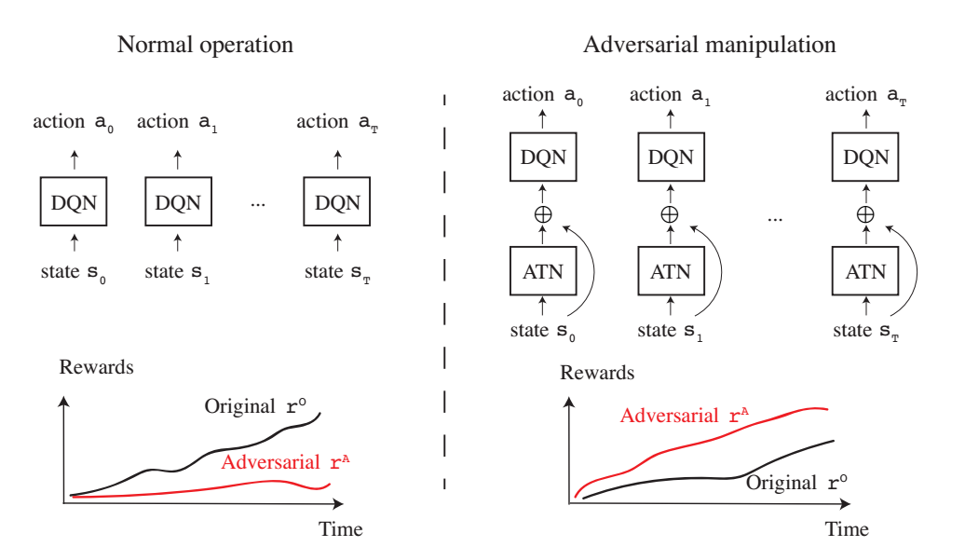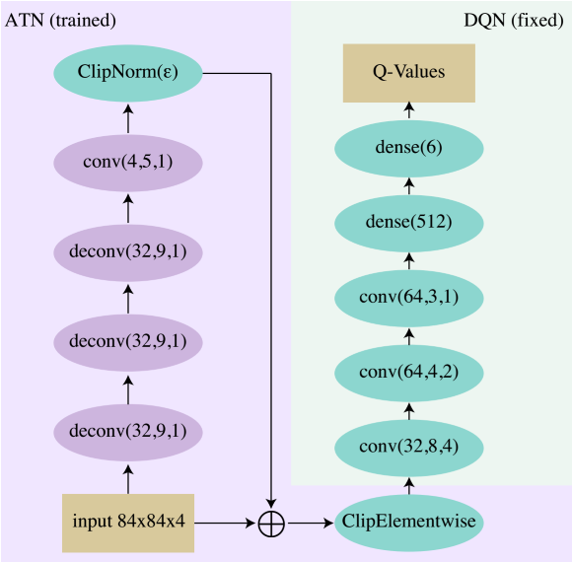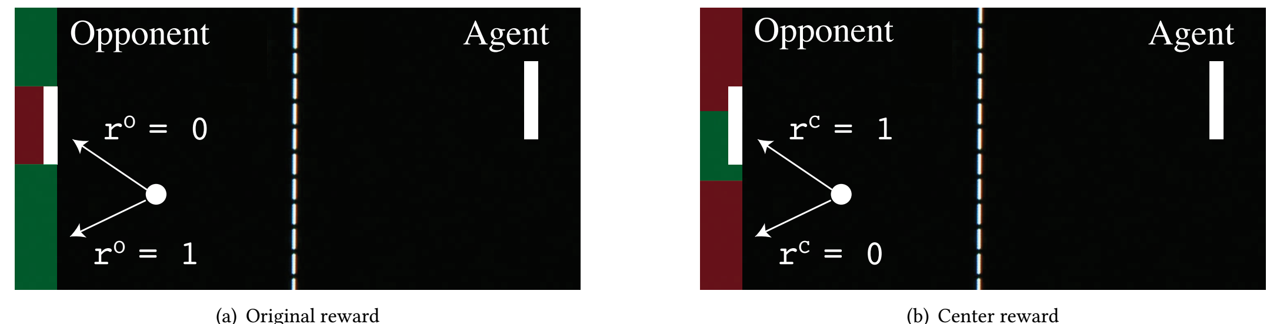# 论文阅读

## Sequential Atacks on Agents for Long-Term Adversarial Goals

Posted by MY on September 25, 2018

# 简介

• 通过在受害者策略网络上j进行一系列攻击达到任意的攻击奖励
• 由于受到攻击，受害者智能体被误导以优化对手的攻击奖励

# 攻击机器学习模型的基本概念

• 分类
• 生成
• 检测
• 分段
• 深度策略网络

## 有目的攻击 vs 无目的攻击

• 无目的攻击：使得模型的预测出现错误
• 有目的攻击：使得模型的得到某种指定的错误预测结果

## 攻击算法类型

• 计算目标网络$f$的梯度$\triangledown xf(x)$
• ATN是一个学习函数$g$，它将输入$x$变换为对抗扰动$g(x)$，使得当给出输入$g(x)$时受害者网络$f$被欺骗
ATN可以定义为如下网络：&g_{f,\theta}(x):x\in X\rightarrow x’& 其中$\theta$是$g$的一个参数向量，$f$是根据分类标记来输出概率分布的目标网络，$x’\sim x$但$argmaxf(x)\neq argmaxf(x’)$。
我们通过优化如下公式来得到$g_{f,x}$: &argmin_{\theta }\sum_{x_{i}\in X}\beta L_{x}(g_{f,\theta }(x_{i}),x_{i})+L_{y}(g_{f,\theta }(x_{i}),f(x_{i}))& 其中$L_{x}$是输入空间中的损失函数，$L_{y}$是$f$的输出空间上的损失函数以避免学习到特征函数，$\beta$是平衡两个损失函数的权重。

# 与其他方法的比较

## 与Lin的方法比较

• Lin的Enchanting Attack中，对手在时间步$t = 0,\cdots,H-1$时对输入状态（帧）$s_{t}$进行扰动，以在时间$t = H$时引导智能体达到预定义的对抗状态$s_{A}$
• 本文的攻击是使得受害者智能体达到一个对手的攻击奖励$r_{A}$

• 在Behzadan的方法中，对手使用对抗奖励$r_{A}$训练DQN来制造扰动。 并且他们在训练期间应用攻击，以使智能体学习对抗奖励
• 本文的攻击训练了一个前馈状态扰动模块，该模块将在测试时使用，并且不会为规划动作来额外建模一个DQN

# 攻击模型

## 模型概览## 模型结构ATN得到输入帧，以前馈方式计算扰动，并在输入上添加扰动。被扰动的输入被馈送到受害者DQN。在训练期间，DQN参数被固定（绿色椭圆形），而ATN参数被更新（紫色椭圆形）。卷积参数分别表示过滤器、内核大小和步幅，而密度参数表示特征。
对于ClipNorm层，我们使用ε对p进行参数化，使得p = 84·84·4·ε： [\begin{equation} clip_{p}(x) = \begin{cases} \frac{p\cdot x}{\left | x \right |_{2}},& \text{if } \left | x \right |_{2}\geqslant p \
x,& \text{otherwise} \end{cases} \end{equation}] 　　对于ClipElementwise层来说，它是为了将输入限制在$[0,1]$之间： &x \mapsto min(max(x,0),1)&

# 实验

## Pong游戏

-1,& \text{if the ball leaves the frame on the agent’s side,} \
0,& \text{otherwise.} \end{cases} \end{equation}] 攻击奖励 [\begin{equation} r^{O}(s_{t}) = \begin{cases} 1,& \text{if the ball hits the centre 20% of the enemy line,} \
0,& \text{otherwise.} \end{cases} \end{equation}]## 结果

##### Share